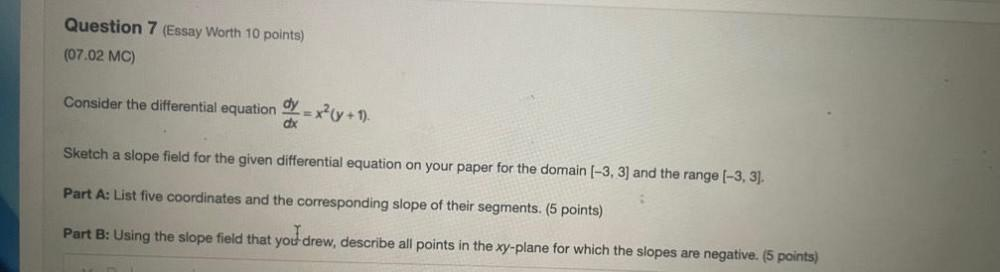Question:

# Consider the differential equation dy/dx = x²(y + 1). Sketch a slope field for the given differential equation on your paper for the domain [-3, 3] and the range [-3, 3]. (A) List five coordinates anConsider the differential equation dy/dx = x²(y + 1). Sketch a slope field for the given differential equation on your paper for the domain [-3, 3] and the range [-3, 3]. (A) List five coordinates and the corresponding slope of their segments. (B) Using the slope field that you drew, describe all points in the xy-plane for which the slopes are negative.